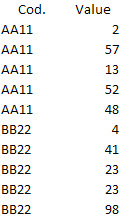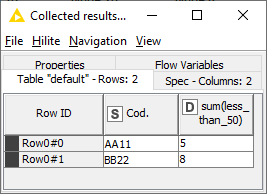# Testing a condition in a moving row-range and store result in a variable (or somewhere else).

Hello all,

I’m struggling with some procedures all day long, and I almost gave up. That’s why I’m here.
I have a 2Million row table that has some information about Revenue, by month, year, etc.

But to illustrate, I will give you this example.
In this case, I have two groups of values that I need to test, in a group of 3, of one of them is less than 50, for example.But, in the next iteration, I cannot jump to another set of 3 values, I just need to move 1 row and test the new group again. Like this:

If one of the values is less than 50, in this case, I need to store a cumulative index for each “cod” group and show how many set has a value less than 50. Like this:

Is there a way to get this? Test a chunk of data, but moving row by row, testing each new group?
Any ideas?

The Window Loop Start node will allow you to define a window size of 3 rows and a step size of 1 row.

Do you already have ideas about the “testing” part of the workflow?

1 Like

I will give it a try, figuring out how to use it and extract the info that I need.
About the testing part, I was thinking about using Column Expressions Node, creating a vector with those three values, and testing if one of them is less than 50 (in my example). If yes, sum up 1 to a variable. In the end, return the variable.

Do you have any suggestion?

Just one more question…
I need to run the window loop in a subgroup (“Cod.” in my example), so I won’t mix values. I need to create a loop within a loop, I guess. Am I right?

Again, many many thanks

Yes, I managed to get this working using a loop within a loop.I took a different approach to you.

My workflow:

1. Evaluates whether each number in the Value column is less than 50. If true, places a test value of 1 in a new column called less_than_50
2. Starts a loop using groups based on the Cod. value
3. Starts a loop based on a 3 row window
4. Sums the numbers in the less_than_50 column for each window, putting the result in a column called sum(less_than_50)
5. Sums the numbers in the sum(less_than_50) column for each Cod. group

Hope it helps!

3 Likes

@elsamuel, thank you! It worked like a charm.
I had no idea the power of loops. I will try to study this.

Thank you!

1 Like

This topic was automatically closed 7 days after the last reply. New replies are no longer allowed.